﻿ On the Internet Traffic Classification: a Multi-criteria Decision Making Approach

### On the Internet Traffic Classification: a Multi-criteria Decision Making Approach

Ihab Sbeity, Bassem Haidar, Mohamed Dbouk

Journal of Computer Sciences and Applications

## On the Internet Traffic Classification: a Multi-criteria Decision Making Approach

Ihab Sbeity1,, Bassem Haidar1, Mohamed Dbouk1

1Math Departement, Faculty of Sciences, Section I, Lebanese University, Lebanon

### Abstract

Traffic classification is a process which categorizes computer network traffic according to various parameters into a number of classes or applications. The interest of internet traffic classification methods has greatly increased over the last decade. The classification methods based on the port number, or based on the payload, suffer from a number of problems, such as the dynamic port allocation and the encrypted applications. For these reasons, new approaches have been proposed without the need to know the port number, typically centered on the statistical behavior of the traffic. In this paper, we develop a novel approach based on multi-criteria decision making methods that achieves a higher significant filtering on the traffic parameters in order to obtain more accurate classification results.

• Ihab Sbeity, Bassem Haidar, Mohamed Dbouk. On the Internet Traffic Classification: a Multi-criteria Decision Making Approach. Journal of Computer Sciences and Applications. Vol. 4, No. 1, 2016, pp 20-26. http://pubs.sciepub.com/jcsa/4/1/4
• Sbeity, Ihab, Bassem Haidar, and Mohamed Dbouk. "On the Internet Traffic Classification: a Multi-criteria Decision Making Approach." Journal of Computer Sciences and Applications 4.1 (2016): 20-26.
• Sbeity, I. , Haidar, B. , & Dbouk, M. (2016). On the Internet Traffic Classification: a Multi-criteria Decision Making Approach. Journal of Computer Sciences and Applications, 4(1), 20-26.
• Sbeity, Ihab, Bassem Haidar, and Mohamed Dbouk. "On the Internet Traffic Classification: a Multi-criteria Decision Making Approach." Journal of Computer Sciences and Applications 4, no. 1 (2016): 20-26.

 Import into BibTeX Import into EndNote Import into RefMan Import into RefWorks

123
Prev Next

### 1. Introduction

The Internet is constantly evolving in scope and complexity, much faster than our ability to characterize, understand, control or predict its behavior and events. The variety and complexity of the modern Internet traffic surpasses the imagination of the Internet architecture designers .

In front of the extensive evolution of the Internet, it is no doubt crucial for service providers to determine the type of the traffic passing through theirs networks, and being consequently able to early discover suspected flow or intruders and avoid prospective damages or troubles. Moreover, it might be suitable to classify the Internet traffic in order to apply statistical –commonly commercial-purpose- studies. This process is well-known as Internet traffic classification [6, 10, 17].

Considering the packet port number to determine the class to which the packet belongs is no more feasible, although the oldest classification methods based on the port number is considerably fast and efficient . Some current applications especially peer-to-peer file sharing do not use a fixed port number in order to hide their identity, and assign port dynamically using some well-known ports of other application. On the other hand, despite the common parlance that ports are no longer useful in identifying application, port-based tools such as Coral-Reef still achieve high precision and recall (> 90%) for several legacy applications and protocols such as DNS and SSH, but Coral-Reef fails to yield accurate classification results with other ephemeral-port applications such as P2P and FTP . Nevertheless, service providers have to respect and protect the privacy of their customers. New algorithms have been developed to support network operations in accordance with user privacy . With this new algorithm, the port-based tools are inexpedient.

Since, a lot of traffic classification approaches have been presented without the need to know the port number of the flow packets. For an excellent review of these approaches, the reader may refer to [3, 11, 17]. These new approaches have basically considered statistical and machine learning techniques [9, 12], or have been based on the packet inspection . The granularity and the computational cost may differ from one approach to another. Nonetheless, the use of specific multi-criteria decision making (MCDM) methods to refine the flow statistics is not yet considered, although the approach seems to be accurate and promising.

Multi-criteria decision making (MCDM) methods  are concerned with structuring and solving problems involving multiple criteria. Typically, MCDM takes as input a certain number of alternatives and criteria, and gives as output the "best" (or the most preferred) alternative according to the set of criteria; in other words, the optimal alternative with the highest degree of desirability with respect to all relevant criteria. A decision matrix initially presents the values of each alternative corresponding to the set of criteria. Intuitively, MCDM may be considered to classify the flow, by looking among a set of available applications, for the best application that a packet may belong to. It is easy to deduct that the set of criteria represents the packet parameters (packet length, data length, duration, etc.).

The purpose of this paper is to present an original traffic classification approach based on MCDM methods, more specifically the Analytical Hierarchy Process (AHP) , and the Technique for Order Preference by Similarity to Ideal Solution (TOPSIS) . AHP will be used to consistently construct the decision matrix basing on pre-known statistical informations or data concerning the applications’ packets. TOPSIS will solve the decision matrix in order to obtain the ideal solution –the best application that a packet may belong to. In order to acquire a set of convenient statistical information, the use of Gaussian distribution and the Gaussian Mixture Model  is vital.

Hence, our MCDM traffic classification process takes as input a set of applications (HTTP, DNS, SSL, FTP, etc.) that will compose the alternatives of the decision making problem. For a given packet P characterized by a certain number of parameters, the process will determine to which applications P does belong. The construction of the decision matrix is done by AHP based on a set of statistical data mostly analyzed basing on the Gaussian Mixture Model (GMM) method . Then, TOPSIS will solve the decision matrix in order to obtain the best solution. Our approach differ from previous classification approaches by the high level of statistical data filtering that promises to deliver higher classification precision.

The rest of the paper is structured as follows: Section 2 recalls the MCDM principles. In particular, we present the basic concept of AHP and TOPSIS. In Section 3, we describe our classification process. As mentioned above, the process is based on three crucial procedures: the pre-known statistical data analysis using Gauss distribution and the Gaussian Mixture Model (GMM) fundamentals, and the two MCDM methods: AHP and TOPSIS. We present how the statistical data traffic is analyzed in order to be provided latterly to AHP to construct a consistent decision matrix. In Section 4, our approach is applied on a case study: preliminary numerical results are shown. Section 5 concludes our paper and describes our ongoing works.

### 2. Overview on MCDM Methods

Doubtless, the most everlasting intellectual challenge in science and engineering is how to make the optimal decision in a given situation. This is a problem as old as mankind. Accordingly, Multiple Criteria Decision Making (MCDM) [15, 16] has been one of the fastest growing problem areas during the last two decades. It aims to make choices in the presence of multiple conflicting criteria. MCDM has become one of the most important and fastest growing subfields of Operations Research.

A MCDM problem is defined by a set of N alternatives and a set of M criteria, and it is usually presented in matrix format, as it is shown by Figure 1. A decision matrix (N×M) is associated to each MCDM problem, in which element aij indicates the performance or preference value of alternative Ai when it is evaluated in terms of criterion Cj. In addition, a weight wj is associated to each criterion Cj, and that represents the relative performance of the decision criterion.

Definition 1: Let A = {Ai , for i = 1,2,3,... ,N} be a (finite) set of decision alternatives and C = {Cj , for j = 1,2,3,..., M} a (finite) set of criteria according to which the desirability of an alternative evaluated. A MCDM problem lies to determine the optimal alternative A* with the highest degree of desirability with respect to all relevant criteria.Download asVeiw figureFigures index
Figure 1. Typical decision matrix

There are many MCDM methods available in the literature. In this work, we focus on the combination of two methods: the Analytical Hierarchy Process (AHP)  and the Technique for Order Preference by Similarity to Ideal Solution (TOPSIS) . To help people make optimal decisions, scholars in the discipline of multiple criteria decision making (MCDM) continue to develop new methods for structuring preferences and determining the correct relative weights for criteria . The most widely used method that permits to construct a consistent decision matrix is AHP. On the other hand, TOPSIS determines the optimal solution defined as the shortest distance from the ideal solution and the farthest distance from the negative-ideal solution in a geometrical sense. Adopting TOPSIS is motivated by the use of the "distance" that makes a significant sense in our classification process as it will be presented in the next section. Moreover, Combining AHP and TOPSIS is encouraging as it has been successfully studied in many previous research works [1, 2].

2.1. AHP

The Analytic Hierarchy Process (AHP), introduced by Thomas Saaty , is an effective tool for dealing with complex decision making, and may aid the decision maker to set preference values and consequently make the best decision. By reducing complex decisions to a series of pairwise comparisons, and then synthesizing the results, the AHP helps to capture both subjective and objective aspects of a decision. In addition, the AHP incorporates a useful technique for checking the consistency of the decision maker’s evaluations, thus reducing the bias in the decision making process.

In order to help the pairwise comparison, Saaty created a nine-point scale of importance between two elements given in Table 1.

#### Table 1. AHP scaleDownload asPowerPoint Slide

Veiw figure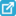View current table in a new window

AHP uses the scale in order to construct a consistent decision matrix that may be latterly solved by MCDM method such as TOPSIS. In order to construct the decision matrix, the basic AHP procedure is summarized by the following two steps:

Step 1: Developing the weights of criteria

The weights may be calculated by a sequence of pair-wise comparison of the criteria, that reflects the importance of criteria each to other. First, a single pair-wise comparison matrix is developed as it is shown in Figure 2.Download asVeiw figureFigures index
Figure 2. AHP – pair-wise comparison between criteria

The value of xij is deducted from the above Saaty’s preference scale; If the criterion Ci is preferred to the criterion Cj, then the value of xij is the preference level and it is in the range {1...9}.

Given the pair-wise matrix, the weights may be calculated by following the stages bellow:

• Sum the values in each column of the pairwise comparison matrix (Figure 3-a).

• Divide each value in a column by its corresponding column sum to normalize preference values. The weights are then the average values in each row (Figure 3-b).

In our traffic classification, note that we will consider the packet parameters as the set of criteria. Obviously, an important question presents here: “how to determine the preference level between two parameters?”. The answer to this question is found in section 4, where we show how it is possible to develop a pair-wise comparison by analyzing a training set (population) of available pre-classified packets.

Step 2: Developing the preference ratings for each alternative for each criterion

For each criterion Ck, a pair-wise comparison matrix is developed, containing the pair-wise comparisons of the performance/preference of alternatives on each criterion. In Figure 4, the value q(k)ij represents the preference level of alternative Ai relatively to alternative Aj according to the criterion Ck. The value of q(k)ij is as well deducted from Saaty preference scale (q(k)ij {1..9}).Download asVeiw figureFigures index
Figure 3. AHP - Developing the weights of criteria

Following the same stage as the weights development of the AHP method, the preference values aik (for i= 1…N) of the alternatives on the criterion Ck are generated, and this, for all the criteria. Thus, the preference rating of alternatives is developed.

Again, an important challenge remains to define the consistent way to obtain the value of q(k)ij. For a giving packet parameter (criterion), finding the preference value between two applications (alternatives) is a crucial issue addressed in section 4.

In addition, we mention that AHP also incorporates a useful technique for checking the consistency of the decision evaluation. A consistency test may be applied to our study to verify if the considered pairwise comparisons are reliable. For further information about the consistency test, refer to .Download asVeiw figureFigures index
Figure 4. AHP – Developing the alternatives’ preference values
2.2. TOPSIS

TOPSIS (Technique for Order Preference by Similarity to Ideal Solution) is one of the useful Multi Attribute Decision Making techniques, and is very simple and easy to implement. Therefore, it is used when the user prefers a simpler weighting approach.

TOPSIS method was firstly proposed by Hwang and Yoon . According to this technique, the best alternative would be the one that is nearest to the positive ideal solution and farthest from the negative ideal solution. The positive ideal solution is composed of all best values attainable of criteria, whereas the negative ideal solution consists of all worst values attainable of criteria. In this study, TOPSIS method is used to determine the application (best alternative) to which a packet does most likely belong.

In the following, we recall the steps followed by TOPSIS to find a solution. The input of TOPSIS process is the decision making formed by AHP, and its output is the best alternative:

Step 1: Normalization of the decision matrix

The normalization process consists on dividing the element of each column on the decision matrix, i.e. aij by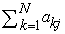. The obtained matrix is a normalized decision matrix.

Step 2: Construct the weighted normalized matrix

It consists on multiplying each column j of the normalized decision matrix by its associated weight wj. In this matrix, the value of row i and column j is denoted vij

Step 3: Determine the ideal and negative ideal alternatives

Let J be the set of benefit attributes or criteria (more is better), and J' be the set of negative attributes or criteria (less is better). The ideal alternative A* is an artificial solution that contains the ideal value of each alternative for each criterion. in other words, A* = { v1* , …, vn*}, where vj* ={ max (vij) if j J ; min (vij) if j J' }.

Similarly, the negative ideal alternative A’ contains the worst value for each alternative for each criterion.

Step 4: Calculate the separation measures for each alternative.

For each row i that corresponds to the alternative Ai, the separation from the ideal Si*, and the separation from the negative ideal alternative S'i, are given by: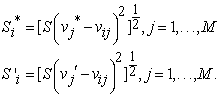Step 5: Calculate the relative closeness to the ideal solution

For the alternative Ai, the relative closeness to the ideal solution Ci* is defined as:The alternative with Ci* closest to 1 is the best alternative following TOPSIS. In our case study, TOPSIS will return the application to which an internet packet does belong.

### 3. The MCDM Traffic Classification Process

Giving an Internet packet P characterized by a certain number of parameters, and a training set of existing applications, the problem consists on determining to which application the packet P belongs. Note that a packet P is defined by M affecting parameters (pr1, pr2, .. , prM) and we look, among N applications (AP1, AP2, .., APN), to which application does P belong. We consider that the alternatives of the decision matrix of Figure 1 (A1, .., AN) represent the available applications (AP1, AP2, .., APN), and the criteria (C1, … , CM) are the packet parameters (pr1, pr2, .. , prM). Therefore, in the following we will use the notations Ai to denote the application APi and Ck to denote the parameter prk.

Our classification process is described by Figure 5.Download asVeiw figureFigures index
Figure 5. MCDM traffic classification process

First, the process requests a statistical study on a population related to the available applications to determine a training set of pre-well-classified packets. The Gaussian distribution concepts and the Gaussian Mixture Model (GMM) are necessary to derive the statistical properties of this population. AHP considers the set of statistical information to create a decision matrix that will be resolved by TOPSIS to determine to which application the packet belongs to. Thus, the basic point in the process is to provide to AHP coherent information that permits to achieve the pair-wise comparisons previously described.

Recall that the input of AHP are the values of the pair-wise comparisons: xij of Figure 3a, and q(k)ij of Figure 4. In the following, we assume the existence of a set G of pre-well-classified packets, and we will look to extract from G the information needed to apply AHP. Note that the set G may be seen as the composition of the N subsets {G1,..., GN}, where Gi contains the packets of type Ai. In addition, we denote by G(k) the cut of G according to the criteria Ck, and let Gi(k) be the subset of value of G(k) related to the set of well-classified packet of type Ai. Figure 6 illustrates the previous notation of subsets.

As it is shown in section 3, AHP operates in two steps: the first step (step 1 of section 3.1) allows developing the weights of criteria, and the second step (step 2 of section 3.1) allows developing the preference ratings of alternatives according to each criterion. By similarity, consider the following two phases to extract the input of AHP: phase 1 consents to provide the coherent information in order to apply step 1 of AHP, and phase 2 provides the information needed to apply step 2.Download asVeiw figureFigures index
Figure 6. Decomposition of the training set G

Phase 1

In this phase, the information about the packet P is not used; only the statistics of the packets of the set G are considered. We aim to develop a score for each (parameter) criterion Ck, denoted by the value Sk. The score Sk reflects how much the criterion Ck is important in the classification process as it allows to distinguish between the alternatives. Typically, more the values of alternatives according to Ck are "scattered", more the criterion is significant (the criterion will then obtain a large score). When the values of alternatives according to a criterion are close, ones to each other, then this criterion will not have a strong discrimination in the classification process. Given the scores of all criteria, the pair-wise comparisons can be easily performed by deducting the values xij of the Figure 3.

To compute the value of Sk, we first consider the following notations. Denote by:

maxk : the maximum value taken by all the packets of G according to the criterion Ck.

mink: the minimum value taken by all the packets of G according to the criterion Ck.

And consequently, we define the Interval Ik = [mink .. maxk], and then we decompose Ik into T equal sub intervals Ik(t) (t = 1 ..T).

For each subset Gi, let λki(t) be the average value of Gi in the sub interval Ik(t). For the interval t, the average distance between two subsets Gi and Gj, i.e. two alternatives Ai and Aj, is then |λki(t) - λkj(t) |. And the overall average distance between two subsets Gi and Gj according to the criterion Ck is given by: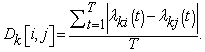The value of Sk is then given by:In other words, the score Sk represents the average distance between the alternatives (applications) according to the criterion Ck (the parameter). For simplicity, we define the distance as mentioned above; however, it may depend of any other sophisticated statistical function.

Now, to generate the values xij (of Figure 3) used by AHP for the pair-wise comparison between the criteria Ci and Cj, a simple function F is applied to the set of scores S = {Sk, k = 1 .. M}, i.e. F : S× S à {1, .., 9}. For any two criteria Ci and Cj, the function F is a simple transition of the value |Si - Sj| into the space {1, .., 9}. The choice of the function F respects the consistency of the decision matrix.

Finally, recall that this phase is fixed once for all the packets subject of classification, and consequently it does not affect the complexity of the classification process.

Phase 2

As it has been described in phase 1, the packet P subject of classification does not influence on the calculation of the weights of the criteria (parameters). Nevertheless, the packet parameters values are essential in the generation of the pair-wise comparison values q(k)ij (Figure 4) used by AHP to calculate the preference ratings for each alternative for each criterion. Accordingly, this phase is applied in the classification process of each new packet.

As it has been shown in Figure 6, considering the training set of pre-well-classified packet G, we denote by G(k) the cut of G according to the criteria Ck, and let Gi(k) be the set of value of G(k) related to the set of well-classified packet of type Ai.

In addition, for a packet P, denote by pk the value corresponding to the criteria Ck, i.e. the parameter prk, of P. For a given criteria Ck, and for the alternative Ai, we aim to develop the value of vi(k) defining how much is it possible for the value pk to belong to the set Gi(k). Again, when these values are developed, the values q(k)ij may be deducted by applying a simple transition F' on the set v(k) = {vi(k); i = 1 .. N}, i.e. F': v(k )× v(k ) à{1, .., 9}. The choice of the function F' respects the consistency of the decision matrix.

We consider that the value vi(k) is the probability density function (PDF) of the distribution of Gi(k) . Therefore, considering the simple case where the distribution of values of the set Gi(k) follows a Gaussian distribution, the value vi(k) is the probability density function (PDF) of the Gaussian distribution, given by:Where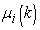and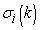represent respectively the mean and the deviation of the distribution Gi(k).

However, an adequate generalization of Gi(k) is to consider its distribution as a GMM (Gaussian Mixture Model). The value of vi(k) is still also the PDF of a GMM given by: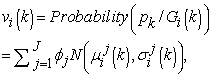Where the distribution Gi(k) is seen as the mixture of J Gaussian distributed components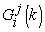, each with its meanand variance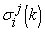. The values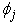are called mixture weights, or prior probability of the component, withThere are many methods to calculate the value of. Note that a variety of approaches to the problem of mixture decomposition -- and consequently the calculation of-- have been proposed, many of which focus on maximum likelihood methods such as expectation maximization (EM) or maximum a posteriori estimation (MAP). For purpose of simplicity, let us consider the value of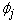to represent the density of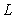over Gi(k) which is equal to [ cardinality ()/ cardinality (Gi (k)) ].

So, all the values vi(k) can be computed whatever the distribution Gi(k). Consequently, to generate the values q(k)ij (of Figure 4) used by AHP for the pair-wise comparison between the criteria, the function F’ may now be applied.

In conclusion, the classification process is accomplished: AHP will produce a decision matrix used by TOPSIS to determine the alternative (application) to which P does belong.

### 4. Preliminary Results

As a primary application of our technique, we have applied the MCDM classification process to the following case study:

• 3 types of applications (alternatives) are considered: DNS, HTTP, and SSL.

• 5 parameters are considered (as criteria), which are: Mean-inter-arrival, Mean-packet-size, Duration, NPackets, and Payload-size.

• The set G of pre-well-classified packets contains the statistics of ≈ 105 packets.

The statistical analysis of the set G shows that the distribution of data according to the two parameters Mean-inter-arrival and Mean-packet-size should be considered as a GMM (Figure 7).

However, the distribution of data according to the parameters NPackets, Payload-size may be easily considered as Gaussian (Figure 8).Download asVeiw figureFigures index
Figure 7. parameters following GMM distributionDownload asVeiw figureFigures index
Figure 8. parameters following Gauss distribution

Considering the previous observations, the MCDM classification process is applied to classify ≈ 108 packets. We have obtained a success rate equals to 96% in a relatively small execution time.

Finally, although the MCDM classification process is applied on a simple case study, but the preliminary results promises to obtain attractive outcomes if the process is used in more sophisticated cases. This point is on the top of our perspectives.

### 5. Conclusion

In this paper, we have developed a new technique in the domain of internet traffic classification. The internet packets are classified into a number of application classes by the mean of two multi-criteria decision making methods: AHP and TOPSIS. The key points in our work are, not only the description of the decision model, but also the way to provide the necessary information needed to apply the decision methods. Our process is based on the knowledge of a set of pre-well-classified packets. The application of our technique on a simple case study gives attractive classification results.

The technique which we propose in this paper will remain immature if it is not applied on more sophisticated case studies with a large mix of application classes and biggest number of packets. Moreover, It is doubtless crucial to achieve a comparative study with other classification techniques in order to underline the weakness and the advantages of our approach. On the other hand, applying our MCDM classification process in a real time case is necessary to determine its reliability, though we strongly believe that our process may be easily used in real time classification as it may be deducted from the primary “static” application of the technique. All the above points are the subject of our ongoing works.

### References

  Ball, Serkan, and Serdar Korukoğlu. “Operating system selection using fuzzy AHP and TOPSIS methods.” Mathematical and Computational Applications 14.2 (2009): 119-130.In article  Dağdeviren, Metin, Serkan Yavuz, and Nevzat Kılınç. “Weapon selection using the AHP and TOPSIS methods under fuzzy environment.” Expert Systems with Applications 36.4 (2009): 8143-8151.In article View Article  Alberto Dainotti, Antonio Pescap´e, and K.C. Claffy. Issues and future directions in traffic classification. Network, IEEE, 26(1): 35 -40, january-february 2012.In article View Article  Finamore, A., Mellia, M., Meo, M., Rossi, D.: Kiss: Stochastic packet inspection classifier for udp traffic. IEEE/ACM Transaction on Networking 18(5), 1505-1515 (2010).In article View Article  Hwang C. L. and Yoon, K., Multiple attributes decision making methods and applications, Springer, Berlin, 1981..In article View Article  PubMed  H. Kim, K. Claffy, M. Fomenkov, D. Barman, M. Faloutsos, and K. Lee. “Internet traffic classification demystified: myths, caveats, and the best practices”. In Proc. of ACM CoNEXT 2008, Madrid, Spain, 2008.In article View Article  Kirby, Alan J., Jeffrey A. Kraemer, and Ashok P. Nadkarni. “Transferring encrypted packets over a public network.” U.S. Patent No. 5,898,784. 27 Apr. 1999.In article  Lindsay, B. G. (1995). Mixture Models: Theory, Geometry, and Applications. NSF-CBMS Regional Conference Series in Probability and Statistics 5. Hayward: Institute of Mathematical Statistics.In article  McGregor, A., Hall, M., Lorier, P., Brunskill, J.: Flow Clustering Using Machine Learning Techniques. In: Barakat, C., Pratt, I. (eds.) PAM 2004. LNCS, vol. 3015, pp. 205-214. Springer, Heidelberg (2004).In article View Article  David Moore, Ken Keys, Ryan Koga, Edouard Lagache, and K. C. Claffy. “The coralreef software suite as a tool for system and network administrators”. In Proceedings of the 15th USENIX conference on System administration, San Diego, California, 2001.In article  T. T. T. Nguyen and G. Armitage. A survey of techniques for internet traffic classification using machine learning. IEEE Communications Surveys & Tutorials, 10(4):56-76, 2008.In article View Article  Roughan, M., Sen, S., Spatscheck, O., Duffield, N.: Class-of-service mapping for QoS: a statistical signature-based approach to IP traffic classification. In: ACM SIGCOMM Internet Measurement Conference (IMC 2004), Taormina, IT (October 2004).In article View Article  Saaty, T.L., The Analytic Hierarchy Process, McGraw-Hill International, New York, NY, 1980In article  Schneider, P.: TCP/IP Traffic Classification Based on Port Numbers. http://www.schneider-grin.ch/media/pdf/diploma_thesis.pdf (20.08.2010), 1996.In article  Triantaphyllou, Evangelos. Multi-criteria decision making methods: a comparative study. Vol. 44. Springer Science & Business Media, 2013.In article  Gwo-Hshiung Tzeng, Jih-Jeng Huang “Multiple attribute decision making: methods and applications.” Multiple Attribute Decision Making: Methods and Applications (2010).In article  S. Valenti, D. Rossi, A. Dainotti, A. Pescape, A. Finamore, and M. Mellia, “Reviewing traffic classification,” in Data Traffic Monitoring and Analysis, 2013, vol. 7754, pp. 123-147.In article  Zimmermann, Hans-Jürgen. Fuzzy set theory—and its applications. Springer Science & Business Media, 2001.In article View Article